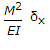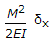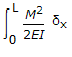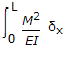# Civil Engineering - Theory of Structures - Discussion

Discussion Forum : Theory of Structures - Section 1 (Q.No. 15)
15.
The total strain energy of a beam of length L, having moment of inertia of its section I, when subjected to a bending moment M, isExplanation:
No answer description is available. Let's discuss.
Discussion:
5 comments Page 1 of 1.

Jayshree odedra said:   11 months ago
The strain energy of a beam due to bending (Ub) having length L and moment of inertia of its section I, when subjected to a bending moment M is given by,

Related Points:

The strain energy of a beam due to axial loading (Ua) having length L and cross-sectional area A, when subjected to an axial load P is given by,

The strain energy of a beam due to torsion (Ut) having length L and polar moment of inertia of its section J, when subjected to a torque T is given by,

Where E is the modulus of elasticity and G is the modulus of rigidity.

Shantanu Sutradhar said:   2 years ago